# Procedural Understanding in Teaching Mathematics

My self-imposed holiday blogging break is over. <cracks knuckles>The holiday break affords teachers the opportunity to reflect. One dimension I think many overlook in teacher reflection is to sit and think deeply about one's content area. I would even be bold enough to say the best math teachers are mathematicians at heart. When I sit down to think about mathematics, as a high school math teacher, I like to approach it like a person that can solve a Rubik's Cube. When you watch this feature below on a speed solver, Chester Liam makes the following claim about speed solving a Rubik's Cube:

As a speed solver? No, there is no math involved, no thinking involved. It's just finger dexterity and pattern recognition. There is nothing, no thinking involved in the entire solving process. [1:02 - 1:14]

We can unpackage Chester's thoughts about the Rubik's Cube and how he solves it so quickly by applying mathematical structure to the cube. However, we should ask: what is the objective? What are we trying to do? There's gobs and gobs of mathematics wrapped up in speed solving a Rubik's Cube, but if Chester were to pay attention to the procedures he is applying, it would inhibit his ability to solve the cube quickly.

But consider this: what if Chester attempts to teach how to solve the cube to another person? What would he have to do? What examples, explanations, and demonstrations would he utilize to teach his pupil speed solving? What implications does this thinking have on teaching mathematics? There would be many features of speed solving the learner may not perceive until Chester brings it to the learner's attention. And if Chester's choices are calculated, deliberate, purposeful... the learner may be helped or hindered dependent upon Chester's ability to communicate his thinking which leads to the automaticity of the procedures he applies to solve the cube. Just like learning how to read or learning how to drive a car, we want to teach learners how to do these tasks so well they become 'automated' at some level.  To understand mathematics deeply, I believe it is often necessary to unpackage some of these automated tasks. I will share an example of such a mental exercise below.

Today I've been thinking about procedures we accept as true while doing math at the high school level, algebra in particular. As an example, suppose we want to determine the location of the x-intercept for the line 5x - 3y = 7. We might approach this 'task' in the following way: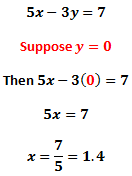And we might even graph the line to confirm our solution...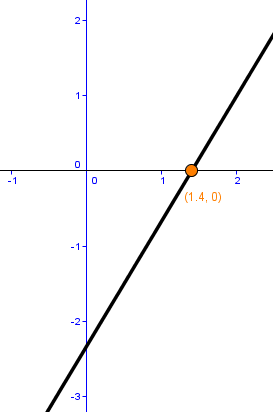Yep. There it is. The x-intercept at (1.4, 0). As a student, we might simply yawn and move on to the next exercise. The student must recognize the y-coordinate of the line will be zero when the line crosses the x-axis. Yes, we have a solution, but I'm not so sure as a math teacher I'm satisfied to stop there. What if we take a different approach? Let's turn the Rubik's Cube and look at the problem another way. A 'typical' algebra student might subscribe to the church of y = mx + b and do the following...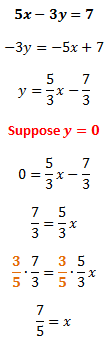Just another equally valid path to the value of the x-intercept. I'd like to focus on something in one of the above lines.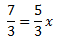This statement says five-thirds of some mystery number is seven-thirds. So, what's the mystery number? I think if we asked a room full of high school math teachers to draw a diagram explaining why we multiply each side of this equation by the multiplicative inverse of 5/3, namely 3/5, we would get some really surprising results. It's not an indictment of teacher education. Rather, it's to say some teachers may not have considered the how's and why's of this procedure before for the same reason someone learning to speed solve a Rubik's Cube may miss a key structure. They may not have the experience of needing to know why it works. Rather, it was more important that they can find the x-intercept of the line; the multiplication by the multiplicative inverse was viewed as "below" the task or level at hand. Perhaps a student never asked "why?" at the critical moment to give the teacher pause.

I struggled to produce the corresponding fraction diagram without trying to reverse engineer the solution. When I write this blog, I often worry I might make a mistake that will be indelibly written into the electronic space of the Internet. But this worry violates the spirit of my blog's theme: that we need to make mistakes in a good direction to evolve our mathematical understanding. Here's the images of my failed by-hand attempts to generate this fraction diagram:Chicken scratches, page 2

I had trouble thinking about making the diagram because 5/3 and 7/3 have the same denominator, so I wrote out some equivalent fractions. Then, I wanted to use "ninths" because that made sense to me in terms of the grid on the graph paper I had cut apart. But, I then realized I would need to cut fifths to find the mystery number, and I was REALLY struggling with trying to free-hand cut fifths with the grid in the background. That led me to coordinatize the points of the polygon. Then I had a problem with relationships between the linear units on the horizontal axis and the area (the fact the polygon is not "one" unit vertically... which is basically the notion of a unit fraction we see emphasized in CCSSM). So I abandoned the paper approach in favor of Geogebra because I could generate better precision and be more efficient with respect to time. <Sorry for the sloppiness of this paragraph, but it does describe my thinking and the mistakes I made.>

Below is an image of the fraction diagram I constructed using Geogebra.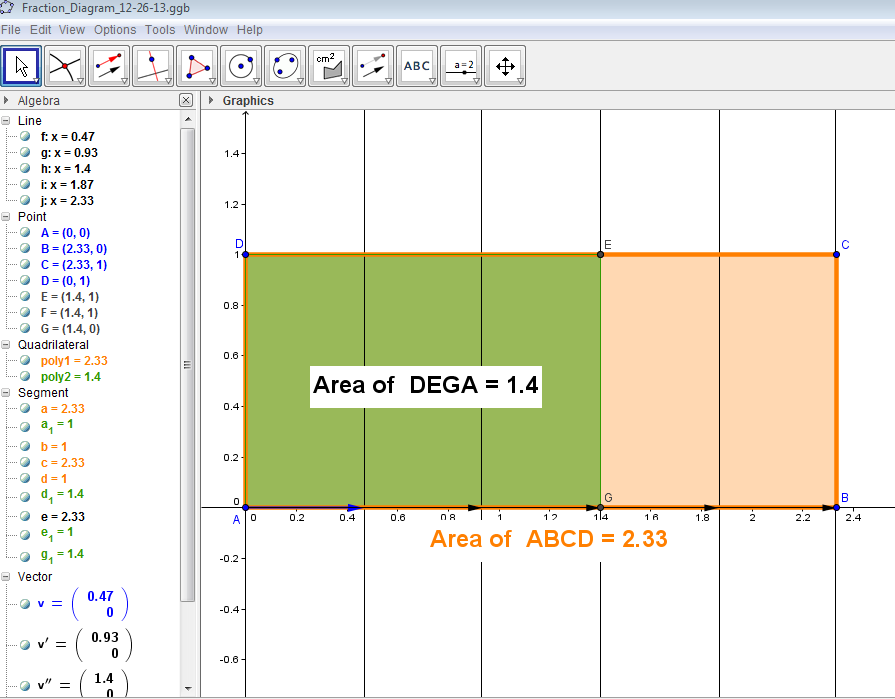When stating the equation, we should think of it as a verbal statement: "Seven-thirds is five-thirds of what mystery number?" Well, if the green polygon represents a whole, then the orange polygon is one-and-two-thirds of that whole. Then the green area of 1.4, which equals 7/5, corresponds to the solution. Mentally, how in the world did I end up with fifths, then? How did I know to cut the horizontal into fifths using vectors and vertical lines in the coordinate plane?

We can think of a fraction in the most basic way. Consider 5/3. If the denominator indicates the number of pieces we partition from a whole, and the numerator indicates how many pieces we possess, then I knew we needed to cut the orange rectangle in a way that would make five pieces. It gets to the root cause of WHY we invert and multiply. The numerator 5 becomes the desired number of pieces, considered in a denominator.

For the sake of time, I will stop my mental exercise there. Between working the problem, generating the fraction diagram by hand and on Geogebra, and typing this up, I've spent about two hours roughly on this article. This professional development is incredibly powerful for me as a teacher, and it's absolutely free (well, not quite free, I do pay for the website hosting, but you get the idea).

Let's end by stirring the discussion pot. Consider our understanding of how to find the x-intercept of the given line. Does our understanding, or lack of understanding, of the fraction diagram and how to construct the fraction diagram (essentially "invert and multiply" in many high school classrooms) inhibit our ability to solve the original problem? Is it still possible to understand the original solution without knowing all the nuts and bolts of the fraction procedure? Stephen Wolfram argues in favor of using computers to automate trivial computation procedures to help us access problems in the world outside school.  How will our teaching of mathematics change as computers continue to become faster and more powerful?

This site uses Akismet to reduce spam. Learn how your comment data is processed.